华中科技大学 985 双一流 211

Huazhong University of Science and Technology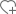关注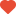已关注•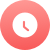1952

•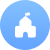公立

•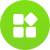综合

•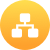教育部

•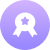本科

•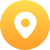湖北-武汉市

•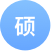214个

•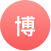183个

院校分数线

多方向招生特色专业院系/专业

• 汉语言文学（本）
• 汉语国际教育（本）
• 哲学（本）

• 公共事业管理（本）
• 政治学与行政学（本）

• 日语（本）
• 德语（本）
• 法语（本）
• 英语（本）
• 翻译（本）

• 生物科学（本）
• 生物制药（本）
• 生物技术（本）
• 生物医学工程（本）
• 生物信息学（本）

• 测控技术与仪器（本）
• 自动化（本）
• 物流管理（本）
• 物联网工程（本）

• 材料科学与工程（本）
• 材料成型及控制工程（本）
• 电子封装技术（本）
• 功能材料（本）

• 通信工程（本）
• 电磁场与无线技术（本）
• 电子信息工程（本）

• 播音与主持艺术（本）
• 传播学（本）
• 广告学（本）
• 广播电视学（本）
• 新闻学（本）

• 给排水科学与工程（本）
• 环境工程（本）
• 建筑环境与能源应用工程（本）

• 电气工程及其自动化（本）

• 金融学（本）
• 经济学（本）
• 国际经济与贸易（本）
• 国际商务（本）
• 金融工程（本）
• 经济统计学（本）

• 飞行器设计与工程（本）

• 信息与计算科学（本）
• 统计学（本）
• 数学与应用数学（本）

• 信息管理与信息系统（本）
• 公共事业管理（本）

• 应用化学（本）
• 化学工程与工艺（本）
• 化学（本）
• 光电信息科学与工程（本）

• 药学（本）
• 中药学（本）

• 能源与动力工程（本）
• 核工程与核技术（本）
• 新能源科学与工程（本）

• 应用物理学（本）
• 物理学（本）

• 光电信息科学与工程（本）
• 微电子科学与工程（本）
• 电子科学与技术（本）
• 集成电路设计与集成系统（本）

• 机械设计制造及其自动化（本）
• 产品设计（本）
• 工业工程（本）
• 测控技术与仪器（本）

• 交通工程（本）
• 土木工程（本）
• 道路桥梁与渡河工程（本）
• 工程力学（本）
• 工程管理（本）
• 交通运输（本）

• 临床医学（本）
• 中西医临床医学（本）
• 医学影像学（本）
• 临床医学（本）
• 口腔医学（本）
• 医学检验技术（本）
• 临床医学（本）
• 临床医学（本）

• 社会工作（本）
• 社会学（本）

• 电子科学与技术（本）
• 微电子科学与工程（本）
• 电子封装技术（本）
• 集成电路设计与集成系统（本）

• 信息安全（本）
• 物联网工程（本）
• 计算机科学与技术（本）

• （本）

• 建筑学（本）
• 城乡规划（本）
• 风景园林（本）
• 环境设计（本）

• 生物制药（本）

• 法学（本）

• 轮机工程（本）
• 船舶与海洋工程（本）

• 市场营销（本）
• 财政学（本）
• 财务管理（本）
• 工商管理（本）
• 物流管理（本）
• 会计学（本）
• 信息管理与信息系统（本）

• 软件工程（本）
• 数字媒体技术（本）

• 水利水电工程（本）

• 预防医学（本）

• 法医学（本）

• 护理学（本）

大学排名知名校友

•李彦宏

•俞洪敏

•撒贝宁

•马云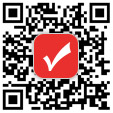400-181-5008

(免长话费 工作日 09:00-17:30)